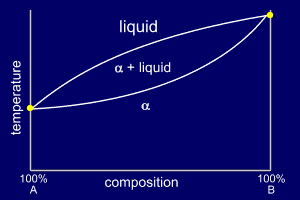# How can I construct binary phase diagrams?

Jun 26, 2015

You use cooling curves to determine the temperatures of the phase transitions for various compositions. Then you plot the points on a graph of temperature vs. composition.

#### Explanation:

A binary phase diagram shows the phases formed in differing mixtures of two substances as a function of temperature.The $y$-axis is temperature, and the $x$-axis is mass percent or mole percent of component $\text{A}$.

You determine the graph experimentally by measuring the cooling curves for various compositions.

Cooling Curves

If you cool a liquid mixture containing about 67% lead and 33% tin (m/m), you get a cooling curve like that below.As the temperature drops, lead freezes out until, at 183°C, both tin and lead are freezing.

Once everything has solidified, the temperature continues to fall.

This gives you two transition temperatures, one corresponding to $\text{liquid → solid + liquid}$, and the other corresponding to $\text{solid + liquid → solid}$.

You repeat this process for various compositions, plot the pairs of points, and join them by smooth lines.

Solid Solutions

If the two substances, such as metals, have similar crystal structures, they often form solid solutions called alloys.

At each end of the phase diagram only pure $\text{A}$ or pure $\text{B}$ is present, and you get a single melting point.

But at compositions in between, you get two phase transitions.

The phase diagram usually looks like that below (α is the solid phase).Eutectic Mixtures

Sometimes two substances can form a mixture that freezes at a lower temperature than any other mixture of the components. This is called a eutectic mixture.

You use cooling curves as before, but the phase diagram looks like that below.